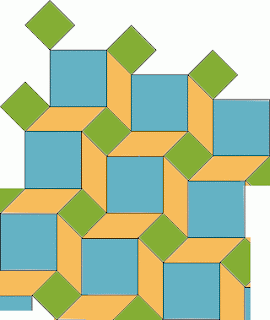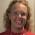Geometry problem: a tiling

UPDATED!

Today I have a geometry problem for you.

Just yesterday I showed you a proportion problem and its solution.

(I want to insert a note here, especially for parents reading this.

Don't feel that you always need to use the problems I present here with your students. Instead, as a homeschooling parent, consider them part of your "math teacher training" that you never got. Reading through problems and their solutions will help you become a better math teacher. Consider yourself an apprentice observing a master completing a task, in this case mathematics problem solving.

It just takes you a minute or two to read this, think it through, try it - and understand (I hope). Your students' or child's book will have similar problems later on when it's time for geometry studies, but if you read these that I present, you will have learned more and can better tackle those problems at that time.)

OK, here we go. Look at the tiling below. Here you can see the tiling in real life, on a floor in Germany.The original problem asks you about the area of the small square and of the parallelogram, given that the area of the large square is 1 square meter and that the ANGLE between the large and the small square is 45°.

But, I simply ask you (or your student) to DRAW this picture. Just take out blank paper, a ruler, and a protractor, and DRAW it. Can you draw it exactly how it is in my picture?

Solution:

This tiling will work (it will tile the plane), no matter what the size of the "little square". So, it is NOT possible for you to draw it exactly like I did, unless you knew something more about the side lengths.

Here's an example where use the same information, but made the small square smaller than in the previous picture:To solve the original problem of finding the area of the little square and of the parallelogram, one would need some more information, such as a side length or ratio of side lengths.

SO, all in all, it's a problem with incomplete information to solve it...

However, someone suggested in the comments a solution to the original problem where we assume that the parallelogram is cut into two right triangles by its diagonal. With that additional information, the area calculation would be solvable.

(BTW, For my first picture, I used golden ratio as the ratio of the side of the large square to the side of the small square.)Anonymous said…
When I try to work this mentally, it seems to me that I need more information. I can imagine the small square as growing or shrinking to almost any size. Have I missed something?Maria Miller said…
That's a good observation, I'd say : )

Just makes me wonder, since I took this from this other site, how did they originally intend for the student to calculate areas? I'm just asking you to DRAW it... and notice what's going on.Eric B said…
If you start divding the parallelogram into right triangles you can calculate the length of the small squares and the height of the parrallelogram. The areaa of both is then easy to find.Maria Miller said…
Well, I checked my drawing. In my drawing, the diagonal of the parallelogram does NOT divide it into two right triangles.

In fact, you can create a tessellation with a small square of any size.

However, it's possible that in the picture from that German floor it is indeed the case that the diagonal of the parallelograms divides it into right triangles. It's just hard to tell from the picture for sure.

That being the case we could solve for the area.I realize I"m late to the punch, but I would also say that in the original drawing it appears that the two larger squares (if the opposite sides of each were to be extended) would cut the smaller square in two, creating two isosceles right triangles, in which case, this could very well be solvable.Maria Miller said…
Tadlewog, I think that the cutting into two isosceles right triangles happens anyway, no matter what the size of the smaller square.Handyman said…
I have been searching for the best designs and patterns that can be used to provide unique look to the space.I am impressed by the information which has been provided here.onegal said…
ok,after studying the original photo of the floor in Germany, this is not that hard to draw. If you simply visualize a straight line running through the center of one large tile, corner to corner, and extending past it, in any of the four possible directions, that line will then form one side of a small square. Using 1/2 inch graph paper helps if you can't visualize the lines. Make the small square 1/2 half inch in size and go from there. The rest falls into place quite easily.this is realy useful, i have been looking a ways to fix my tileing problems. so thanks.